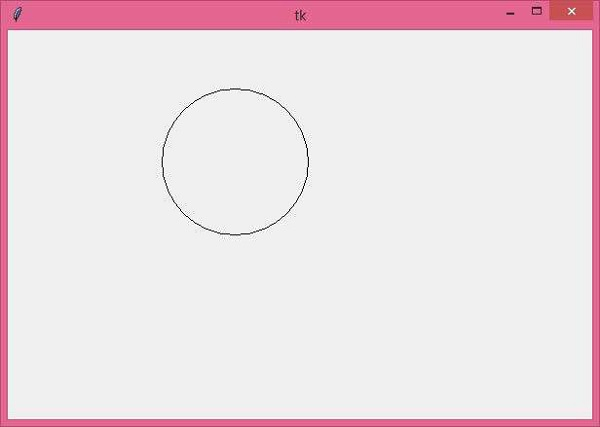# Draw a circle in using Tkinter Python

Tkinter Canvas are generally used for creating shapes such as arc, rectangle, triangle, freeform shapes, etc. All these shapes can be drawn using the inbuilt function available in tkinter library.

## Example

In this example, we will create a Circle using the create_oval(x0,y0,x1,y1) method by passing the following values of coordinates (x0,y0, x1, y1)

#Import the library
from tkinter import *

#Create an instance of tkinter frame
win= Tk()

#Define the geometry of window
win.geometry("600x400")

#Create a canvas object
c= Canvas(win,width=400, height=400)
c.pack()

#Draw an Oval in the canvas
c.create_oval(60,60,210,210)

win.mainloop()

## Output

Running the above code will draw a circle in the canvas. In this example, we have defined the coordinates for (x0, y0, x1, y1) as (60,60,210,210). Thus, it will draw and display a circle in the window.